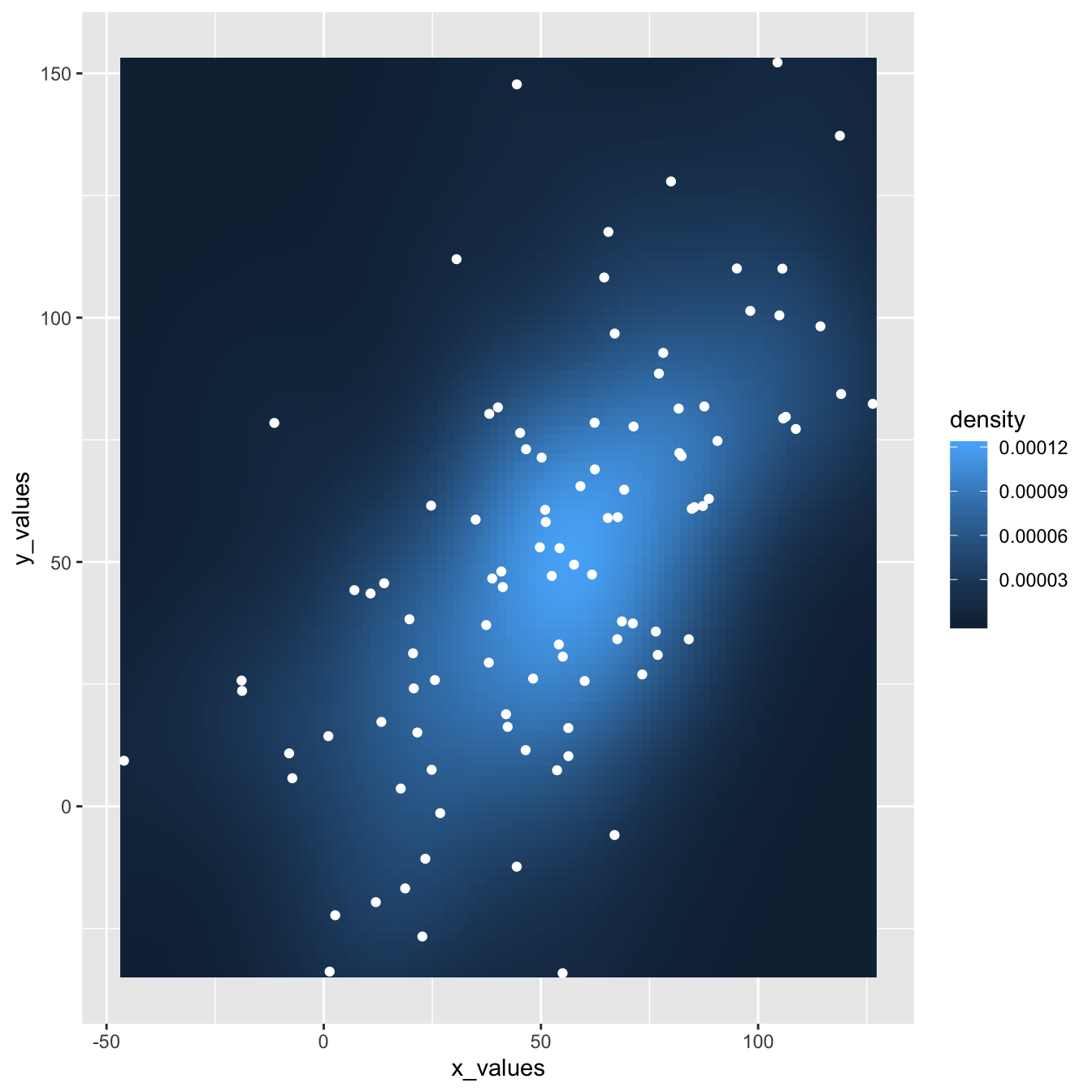# Hexbin chart and scatterplot with ggplot2

This post explains how to build a hexbin chart with a scatterplot on top using `R` and ggplot2. It is an addition to the page about 2d density plot with ggplot2.

This plot extends the concepts described in the 2d density chart with ggplot2 document. It simply illustrates that a scatterplot can be added on top of the 2d density chart.

Thanks Christian Jacob for this submission.``````# library
library(ggplot2)

# data
sample_data <- data.frame(x_values = 1:100 + rnorm(100,sd=20), y_values = 1:100 + rnorm(100,sd=27))

#plot
ggplot(sample_data, aes(x_values, y_values)) +
stat_density2d(geom="tile", aes(fill = ..density..), contour = FALSE) +
geom_point(colour = "white")``````

Related chart types

## Contact

This document is a work by Yan Holtz. Any feedback is highly encouraged. You can fill an issue on Github, drop me a message on Twitter, or send an email pasting yan.holtz.data with gmail.com.